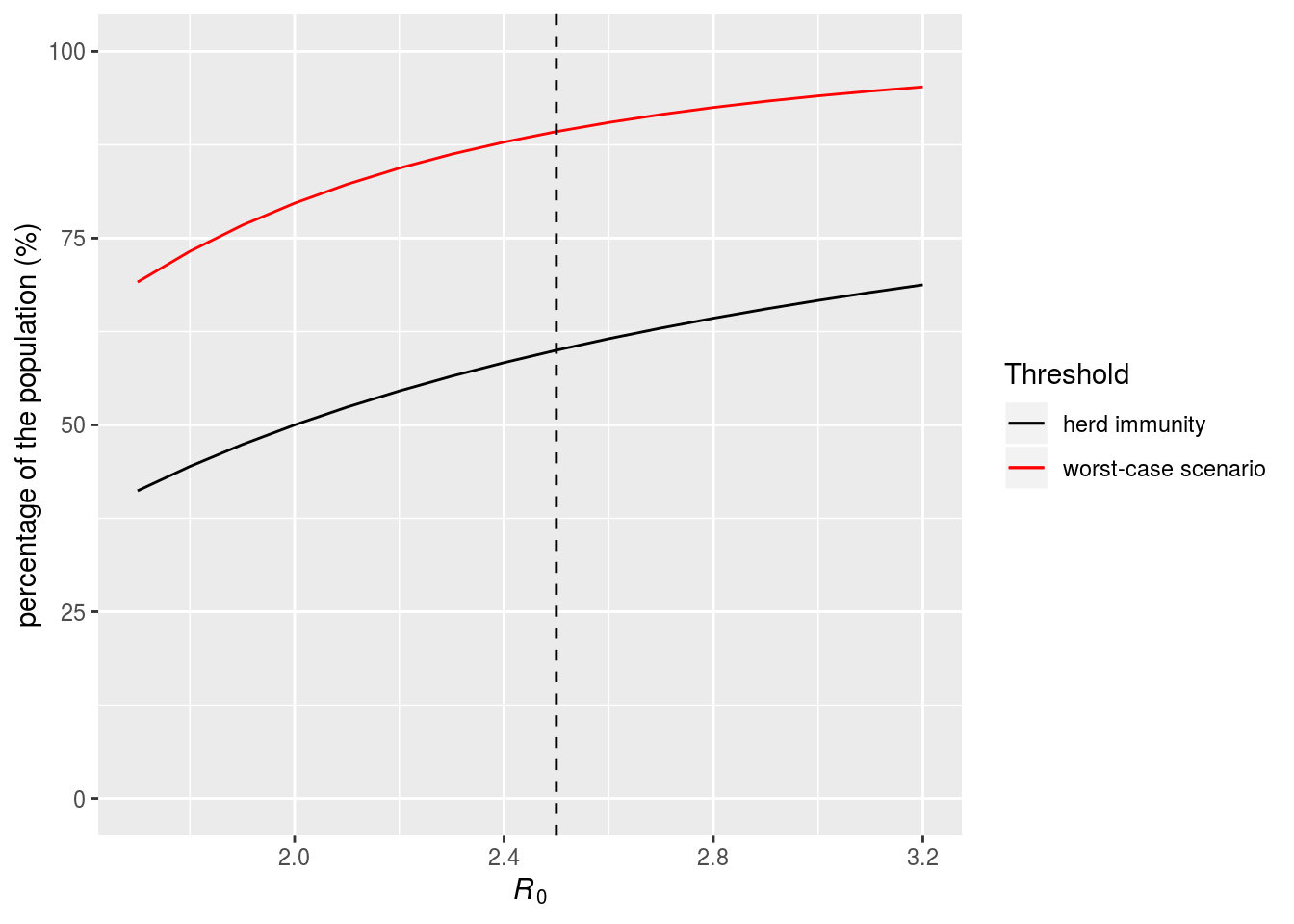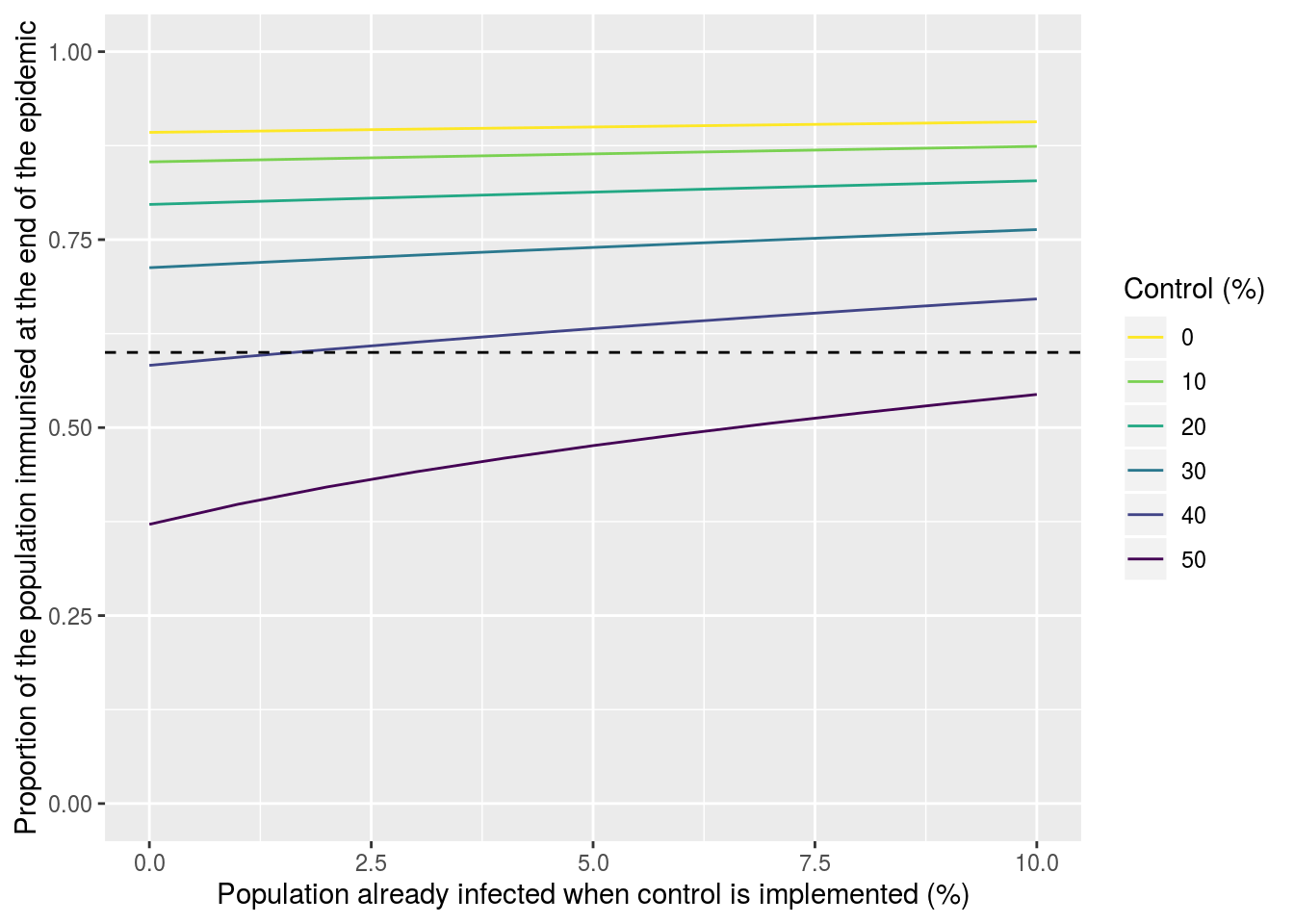# Disclaimer

This text is for research and educational purposes only not a decision-making advice. The following calculations are made using voluntarily simplified assumptions.

For any question, please refer to the French public health authority, Santé Publique France, where one can find all official instructions: https://www.gouvernement.fr/info-coronavirus

The World Health Organisation (WHO) provides as well a comprehensive website: https://www.who.int/fr/emergencies/diseases/novel-coronavirus-2019

# Context

On March 11th, 2020, the WHO announced that the COVID-19 outbreak had reached pandemic stage. On March 12th, the French authorities announced the closure of school structures starting from March 16th. On March 14th, the Prime Minister announced the transition to the third stage of the fight plan against the epidemic and the closure of most of the country’s infrastructure.

For the past few weeks, debates have been taking place regarding the immunization of the population. Indeed, some claim that letting the epidemic grow unchecked is necessary so that herd immunity (also called group immunity) can build up in the population. Conversely, others recommend extremely strict containment measures.

# The basic reproduction number as the epidemic threshold

The basic reproduction number (denoted by $$\mathcal{R}_{0}$$) is the number of secondary infections caused by a contagious individual over his or her infectivity period, while being in a susceptible population. It is a key quantity widely used in epidemiology because an epidemic can only emerge in a population if $$\mathcal{R}_{0}>1$$.

Mathematically, one can write \begin{align} \mathcal{R}_{0} & = \text{transmission rate} \times \text{infectivity period} \times \text{proportion of susceptible individuals} \end{align}

According to this formula, one can see that the less susceptible the population, the lower the risk of emergence. Also, if the whole population is susceptible, then $$\mathcal{R}_{0}$$ depends only on the first two terms. Mathematically, for such an initial value (which we will note $$\mathcal{R}_{0}(t_0)$$), we can calculate the proportion $$p$$ that needs to be immunized to prevent the epidemic, i.e. bring $$\mathcal{R}_{0}$$ below the threshold of 1.

Mathematically, this can be written as: \begin{align} \mathcal{R}_{0}(t_0)~(1-p) & < 1 \end{align}

Expressing this inequality as a function of $$p$$ yields: \begin{align} p & > 1 - \frac{1}{\mathcal{R}_{0}(t_0)} \end{align}

The value of $$p$$ is often referred to as the threshold value for herd immunity to be high enough to prevent the spread of the infection. Intuitively, if a person who is susceptible to COVID-19 is only in contact with immune hosts, that person is indirectly protected from the infection even though he or she is not immune.

As shown in the figure below, the higher $$\mathcal{R}_{0}$$, the higher the threshold for herd immunity. For a virus such as measles, which has an $$\mathcal{R}_{0}$$ greater than 10, it is necessary to immunize more than 90% of the population to prevent an epidemic.

In the case of COVID-19, the $$\mathcal{R}_{0}$$ has been estimated to be around $$\mathcal{R}_{0}(t_0)\approx 2.5$$ in many countries. From this, it follows that if $$1-1/2.5 \approx 60\%$$ of the population is immune, the virus cannot cause an epidemic.

Importantly, this threshold only applies to new epidemics! In other words, once this epidemics of the COVID-19 is finished, if more than two thirds of the French population is immune, we will avoid a new epidemic. This does not mean that the current epidemic will stop once this proportion of the population is immunised, as we will see below.

# Total number of infections

In their paper published on March 6th, Anderson et alii (2020, The Lancet) write:

A simple calculation gives the fraction likely to be infected without mitigation. This fraction is roughly $$1–1/\mathcal{R}_{0}$$. With $$\mathcal{R}_{0}$$ values for COVID-19 in China around 2.5 in the early stages of the epidemic, we calculate that approximately 60% of the population would become infected. This is a very worst-case scenario for a number of reasons.

Unfortunately, their reasoning seems to be incorrect and the worst-case scenario that would be observed in the absence of any control strategies could be much worse.

Simplified models, assuming $$\mathcal{R}_{0}$$ will remain constant during the epidemic, can be used to estimate the total proportion of the population that will be infected following an epidemic wave in the absence of containment measures. These models are simplified because, in practice, the reproductive number does not remain constant as authorities implement measures to contain the spread.

As derived in the seminal article by Kermack and McKendrick (1927, Proc. R. Soc. Lond. A) or recalled in the review article by Hethcote (2000, SIAM Rev), it is straightforward to show that, at the end of the epidemic wave, the final proportion of the population that will have been infected, denoted by $$q^{\star}$$, satisfies the following equation: \begin{align} \mathcal{R}_{0}q^{\star}+\mathrm{log}\left(1-q^{\star}\right) & =0. \end{align}

If the value of $$\mathcal{R}_{0}$$ is known, this equation can be solved numerically (as shown below).

Importantly, this result holds for more relatively detailed compartmental models featuring multiple stages in the natural history of the infection (e.g. a latent stage, an infectious but asymptomatic stage,… – as is observed for COVID-19), which is captured by the analytical formula of $$\mathcal{R}_{0}$$.In the figure above, we can see that the proportion of the population that needs to be immune to prevent the emergence of a new epidemic (black curve) increases with $$\mathcal{R}_{0}$$.

We can also see that this threshold for herd immunity is much lower than the proportion of the population that will be infected if no action is taken (worst-case scenario, in red). In other words, letting the epidemic spread unchecked would lead to a very large proportion of the population being infected.

Finally, in the figure, the vertical dashed line shows the estimated value of $$\mathcal{R}_{0}$$ for France. If $$\mathcal{R}_{0}$$ equals 2.5, in the absence of control measures, approximately 89% of the population would be infected, whereas the threshold necessary to achieve herd immunity (and thus prevent future epidemics) is 60%.

In other words, the difference between Anderson et alii’s worst-case scenario (no control measures) and ours is about 28% (i.e. 18 million infections in France).

# Magnitude of the epidemic with control measures

We will now assume that at a given date (noted $$t_1$$), contact limitation measures are implemented that decrease the initial $$\mathcal{R}_{0}$$ by $$c$$%. The previous formula has to be corrected for the small proportion of the population that has already been infected at $$t_1$$, which will be denoted by $$q_1$$. One can show that the equation to be solved to find the proportion of the population that will have been infected ($$q^\star$$) is now: \begin{align} \left(1-c\right)\mathcal{R}_{0}q^{\star}+\mathrm{log}\left(1-q^{\star}\right)-\mathrm{log}\left(1-q_1\right) & = 0 \end{align}

Assuming that the initial number of recovered (and therefore immune) people is negligible, which can be a strong assumption, we then have two free parameters, which are $$\mathcal{R}_{0}$$ and $$q_1$$.

In the following figure, we represent the total proportion of the population that will have been infected at the end of the epidemic. Each coloured curve represents a more or less intensive control scenario ($$c=0$$ being the worst-case scenario with no control). This proportion of immunized hosts at the end of the epidemic also varies according to the proportion of the population infected at the time where the measures are implemented (the later the action, the more the population is already infected). Finally, the black dashed line indicates the threshold for herd immunity, i.e. the proportion of the population that must be immunized to prevent a new future epidemic.This graph shows that if control is not strong enough ($$c$$ is too low), the infection spreads widely and ultimately affects a much larger proportion of the population than what would have been necessary to prevent the virus from causing further epidemics (the 60% required for herd immunity). Note that the proportion of the population already infected before control implementation has little effect on this outcome for lower control measures, but its impact increases with the insensity of the latter.

We can also see that if the control measures are very strong, the number of infected (and therefore in fine immunised) people remains below the threshold of herd immunity, leaving the population at the mercy of a return of the epidemic as soon as the containment measures are lifted.

An ideal strategy would be to implement strong control until a safe and efficient vaccine is available but this is likely to be unrealistic given the time required for vaccine development.

# Limitations

• The models used in this work are very simple and do not take into account population heterogeneity.

• This approach asummes that individuals can only be infected once within the first epidemic wave. This calculation does not address secondary waves. If immunity is not long-lasting and a secondary wave appears, then $$q^{\star}$$ will increase.

• We do not make predictions regarding the number of deaths in this model for three reasons:

1. It is difficult to estimate what percentage of virus transmission between hosts could be suppressed without endangering the population for other reasons,

2. we do not know at what stage of the epidemic we currently are (the number of people already infected is unknown),

3. the infection prevalence